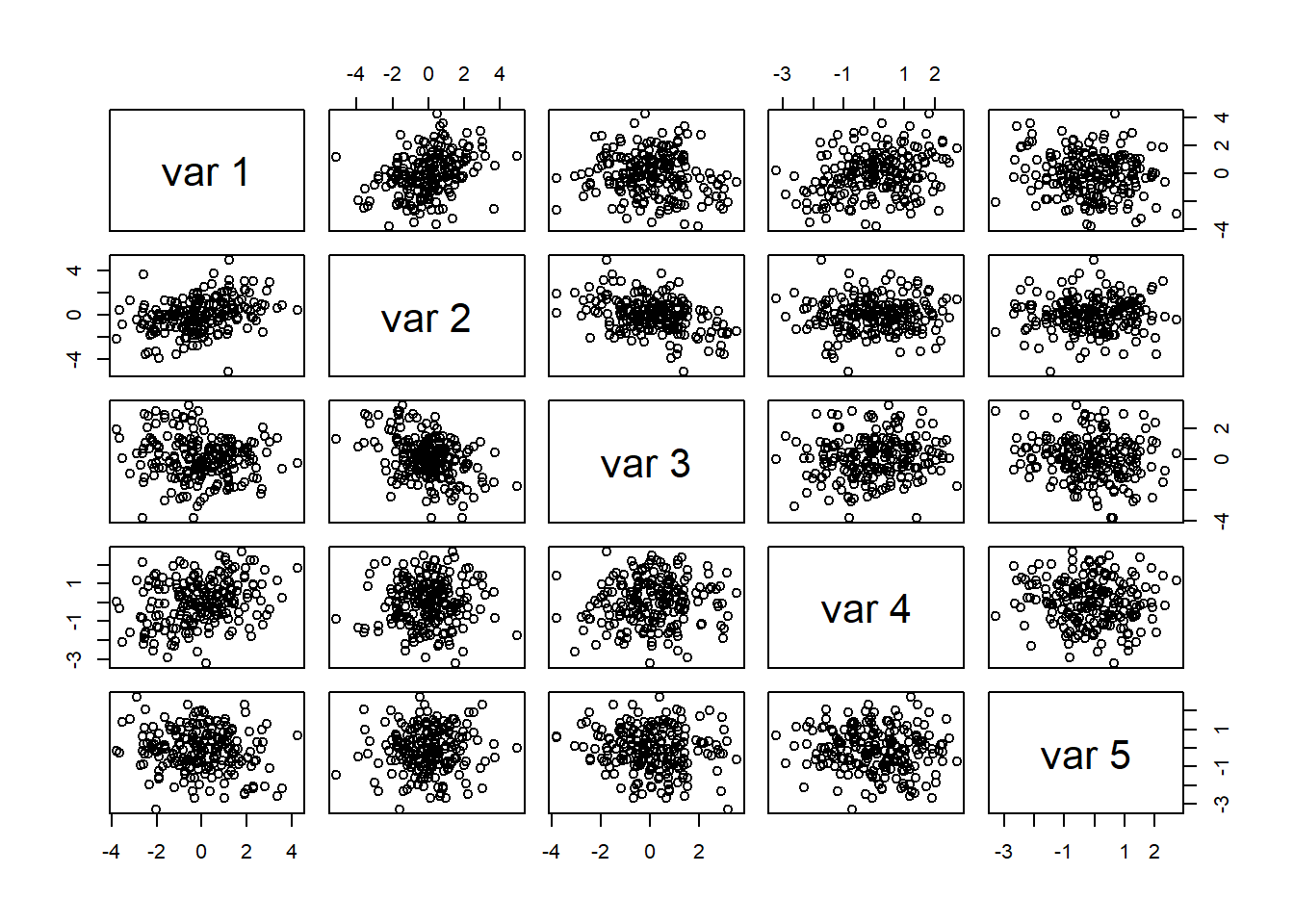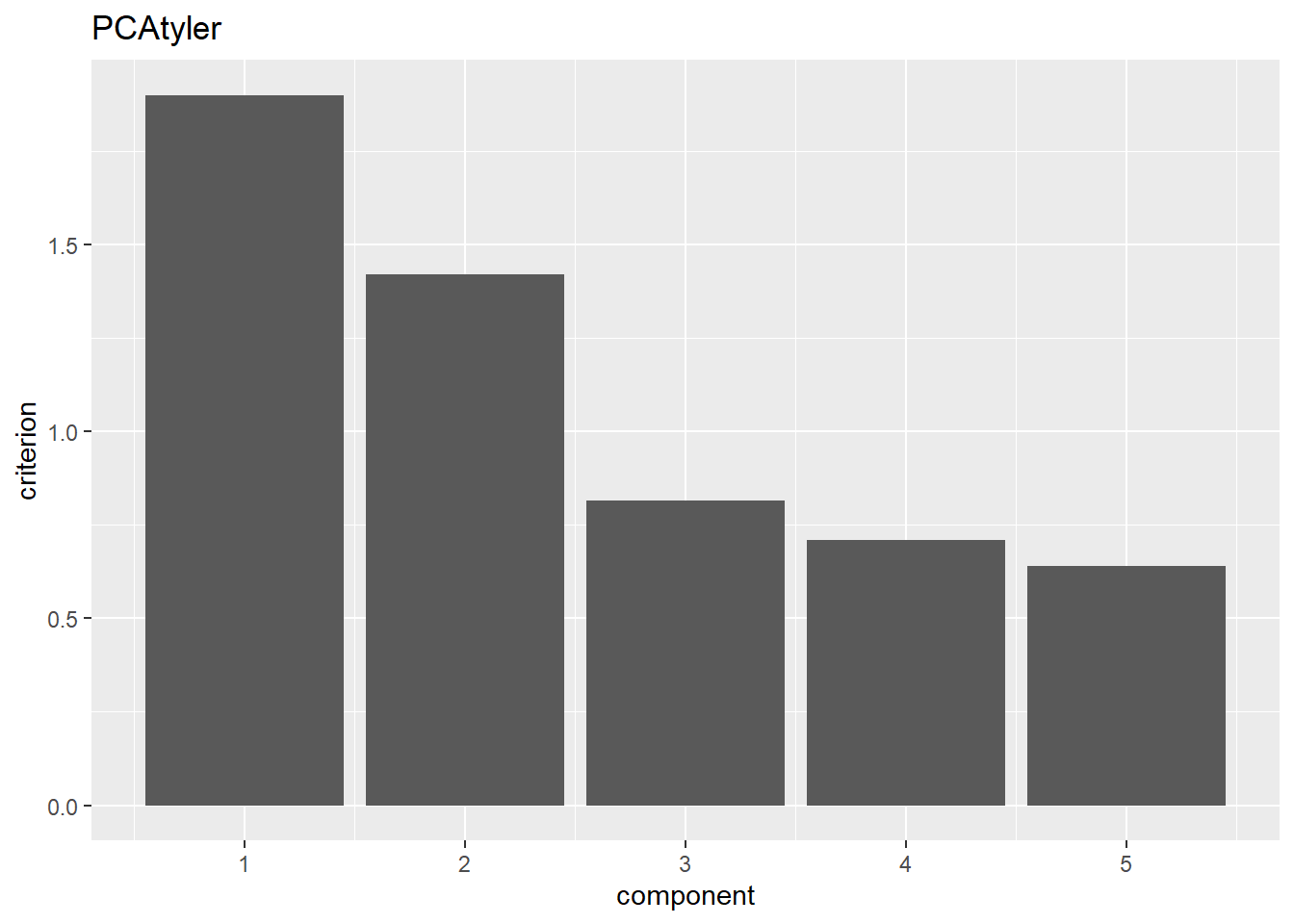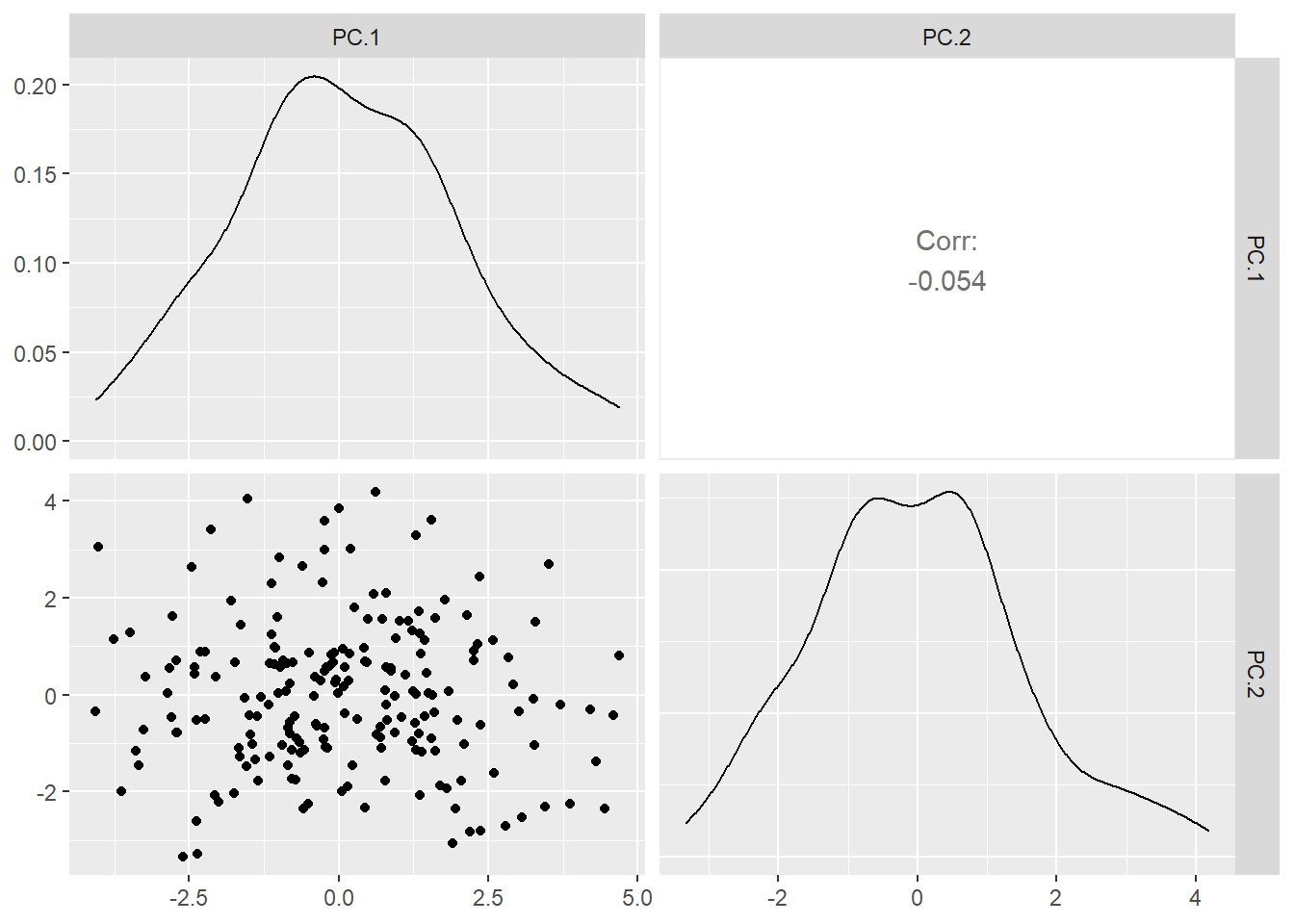## Principal component analysis

In an elliptical model often principal component analysis (PCA) is used as a linear dimension reduction tool and it is assumed that the components with large variation are interesting and the directions with small and equal variation are the uninteresting ones. Note that those components with equal variation form then a subspherical subset.

Assume that $$X$$ is a data matrix with $$n$$ observations and $$p$$ variables and that PCA is performed using a scatter matrix (and location vector) of the user’s choice, such as the regular covariance matrix (and the mean vector).

Then $$X$$ is first centered using the location of choice and, assuming the matrix $$W$$ contains the eigenvectors of the scatter matrix, $$S = XW$$ gives then the principal components where the amount of variation of each component is given by the corresponding eigenvalues $$d_1,...,d_p$$.

The null hypothesis of interest is $H_0: \ d_1 \geq ... \geq d_k > d_{k+1} = ... = d_p.$

### Asymptotic test a specific value of $$k$$

The function PCAasymp offers an asymptotic test for the above hypothesis where the user can choose between two scatter matrices. Using the argument scatter = "cov" centers the data with regular mean vector and performs PCA using the regular covariance matrix. Specifying instead scatter = "tyler" estimates jointly Tyler’s shape matrix and the spatial median using the function HR.Mest from the package ICSNP and uses then them accordingly.

The test statistic is in both cases based on the variance of the last $$p-k$$ eigenvalues: $T = n / (2 \bar{d}^2 \sigma_1) \sum_{i=k+1}^p (d_i - \bar{d})^2,$ where $$\bar{d} = 1/n \sum_{i=k+1}^p d_i$$ and $$\sigma_1$$ is a constant specific for the used scatter matrix and depends on the underlying elliptic distribution and the dimension $$p$$. The value of $$\sigma_1$$ is estimated from the data.

Under $$H_0$$ this test statistic has a chisquare distribution with $$(p-k-1)(p-k+2)/2$$ degrees of freedom.

To demonstrate the function consider the following artificial data:

library(ICtest)
set.seed(1)
n <- 200
S <- cbind(rnorm(n, sd = 2), rnorm(n, sd = 1.5), rnorm(n), rnorm(n), rnorm(n))
A <- rorth(5)
X <- S  %*% t(A)
pairs(X)which means there are two components of interest.

PCAcov <- PCAasymp(X, k=2)
PCAcov
##
##  PCA subspericity test using cov
##
## data:  X
## T = 0.80054, df = 5, p-value = 0.977
## alternative hypothesis: the last 3 eigenvalues are not equal
PCAtyler <- PCAasymp(X, k=2, scatter = "tyler")
PCAtyler
##
##  PCA subspericity test using Tyler's shape matrix
##
## data:  X
## T = 2.1696, df = 5, p-value = 0.8252
## alternative hypothesis: the last 3 eigenvalues are not equal
ggscreeplot(PCAtyler)And in both cases this hypothesis is not rejected.

But for example testing for no interesting component using cov yields:

PCAcov0 <- PCAasymp(X, k=0)
PCAcov0
##
##  PCA subspericity test using cov
##
## data:  X
## T = 126.68, df = 14, p-value < 2.2e-16
## alternative hypothesis: the last 5 eigenvalues are not equal

### Bootstrapping test a specific value of $$k$$

To obtain a bootstrap test for this problem the package offers the function PCAboot. The function can perform the test for any scatter matrix the user has a function for. Important is only that the function returns a list which returns as first element the location vector and as second element the scatter matrix. The function is specified using the argument S and Sargs is an argument where additional arguments to S can be passed in the form of a list.

The test statistic used for the bootstrapping tests is $T = n / (\bar{d}^2) \sum_{i=k+1}^p (d_i - \bar{d})^2,$

and two different bootstrapping strategies are available:

1. s.boot="B1": The first strategy has the following steps:
1. Take a bootstrap sample $$S^*$$ of size $$n$$ from $$S$$ and decompose it into $$S_1^*$$ and $$S_2^*$$.
2. Every observation in $$S_2^*$$ is transformed with a different random orthogonal matrix.
3. Recombine $$S^*=(S_1^*, S_2^*)$$ and create $$X^*= S^* W^{-1}$$.
4. Compute the test statistic $$T^*$$ based on $$X^*$$.
5. Repeat the previous steps $$m$$ times to obtain the test statistics $$T^*_1,...,T^*_m$$.
1. s.boot="B2": The second strategy has the following steps:
1. Scale each principal component using the $$p\times p$$ diagonal matrix $$D^*=diag(d_1,...,d_k,\bar{d},...,\bar{d})$$, i.e. $$Z = S D^*$$.
2. Take a bootstrap sample $$Z^*$$ of size $$n$$ from $$Z$$.
3. Every observation in $$Z^*$$ is transformed with a different random orthogonal matrix.
4. Recreate $$X^*= Z^* {D^*}^{-1} W^{-1}$$.
5. Compute the test statistic $$T^*$$ based on $$X^*$$.
6. Repeat the previous steps $$m$$ times to obtain the test statistics $$T^*_1,...,T^*_m$$.

The p-value is in both cases obtained then as: $\frac{\#(T_i^* \geq T)+1}{m+1}.$

To create the random orthogonal matrices in the bootstrapping approaches the function rorth is used.

To demonstrate how to use another scatter matrix and how to pass on additional arguments we use now the scatter based on the MLE of $$t_2$$ distribution, as for example implemented as the function tM in the ICS package. For that purpose, using strategy two we test using the data from above if $$k=1$$ or $$k=2$$.

PCAtMk1 <- PCAboot(X, k=1, S="tM", Sargs=list(df=2))
PCAtMk1
##
##  PCA subsphericity bootstrapping test using "tM" and strategy B1
##
## data:  X
## T = 23.357, replications = 200, p-value = 0.004975
## alternative hypothesis: the last 4 eigenvalues are not equal
PCAtMk2 <- PCAboot(X, k=2, S="tM", Sargs=list(df=2))
PCAtMk2
##
##  PCA subsphericity bootstrapping test using "tM" and strategy B1
##
## data:  X
## T = 1.417, replications = 200, p-value = 0.8557
## alternative hypothesis: the last 3 eigenvalues are not equal
ggplot(PCAtMk2, which="k")